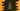# How to read float inputs in C### How to read float inputs in C#:

Many times we need to read the user-input as a floating-point value. For example, if you are taking the user-input to do some mathematical calculations, you need to convert the user-input value to float or integer. Without that, if you try to do any operation like addition, multiplication, etc., it will crash.

So, if we read a value using Console.ReadLine, it will not be converted to a float automatically. Let’s take a look at the below program:

``````using System;

public class Program {
public static void Main() {
float floatValue;

Console.WriteLine("Enter a float value :");
}
}``````

It has a float variable floatValue in this program. It is asking the user to enter a float value. It reads that value using ReadLine() and assigns it to floatValue. But it will throw a Compile time error. Because floatValue is a float variable and Console.ReadLine() returns a string.  We can’t store a string value in a float variable. To solve this error, we need to convert the value to float.

There are different ways we can use to convert a string value to float. Let’s take a look at them:

## Method 1:  By using float.Parse:

We can convert *string *input to float by using this method. float.Parse takes the *string *value as its argument, converts it to float, and returns it. We can pass the return value of Console.Readline() to this method to get the float value.

Now, let’s re-write the above program with float.Parse :

``````using System;

public class Program {
public static void Main() {
float floatValue;

Console.WriteLine("Enter a float value :");

Console.WriteLine("You have entered :" + floatValue);
}
}``````

This program will work. It will give output as like below:

``````Enter a float value :
12.334
You have entered :12.334``````

## Method 2: By using Single.Parse() method :

Single.Parse is another method defined in the Single class that can be used to convert a string to a *float *value. It works similar to the above example. It takes one string as an argument and returns the float value.

We can re-write the above example as like below:

``````using System;

public class Program {
public static void Main() {
float floatValue;

Console.WriteLine("Enter a float value :");

Console.WriteLine("You have entered :" + floatValue);
}
}``````

It will give similar output.

## Method 3: By using Convert.ToSingle() method:

*ToSingle *method of the Convert class works in the same way. We can use it to convert a string to float.

``````using System;

public class Program {
public static void Main() {
float floatValue;

Console.WriteLine("Enter a float value :");